#### Engineering Functions in Excel

Excel offers a wide range of engineering functions that can be used to perform various engineering-related calculations. The built-in Excel Engineering Functions have been provided to perform some of the most commonly used engineering calculations.

In this article, we are going to learn how to use the Excel engineering function.

## CONVERT Function

The CONVERT function in Excel is used to convert a value from one measurement unit to another. For instance, meter to yard.

The syntax for the CONVERT function is as follows:

=CONVERT (number, from_unit, to_unit)

• number – the number you want to convert,
• from_unit – the current unit
• to_unit – the unit into which the value should be converted.

Example:

=Convert (2000,”m”,”yd”)

Explanation: This will convert the value of 2000 from meters to yards.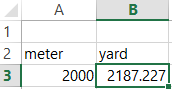The CONVERT function supports a wide range of units of measurement, including units for temperature, weight, length, volume, and time. To see a full list of supported units, you can refer to the Microsoft Excel help documentation.

## IMSUM Function

The IMSUM function in Microsoft Excel is part of the set of “imaginary functions” and is used to calculate the sum of a series of complex numbers.

The syntax for the IMSUM function is as follows:

=IMSUM (inumber1, inumber2…)

where number1, number2, etc. are the complex numbers that you want to sum. Complex numbers are numbers that contain both a real part and an imaginary part, and are often represented in the form a + bi, where a is the real part and b is the imaginary part.

Example:

=IMSUM(“3+4i”, “5-3i”)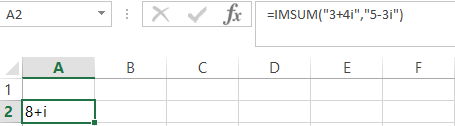It’s important to note that in order to use the IMSUM function, you need to have the “Analysis Toolpak” installed in Microsoft Excel. To install the Analysis Toolpak, you can go to the “File” menu, select “Options,” then select “Add-Ins.” From there, you can choose to install the “Analysis Toolpak.”

## IMREAL Function

Returns the real coefficient of a complex number in x + yi or x + yj text format.

The syntax of the IMREAL Function is:

=IMREAL (inumber)

• Inumber – a complex number for which you want to know the real coefficient.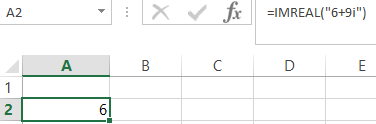Explaination: The true coefficient of 6-9i is 6.

## DELTA Function

Tests whether two values are equal. Returns 1 if number1 = number2; returns 0 otherwise

The syntax of the DELTA Function is:

=DELTA (number1, number2)

• Number1 is the first number and Number2 is the second number. If omitted, number2 is assumed to be zero.

Examples: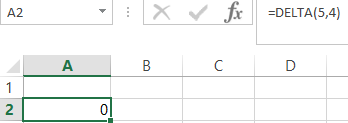Explanation: Number 5 is not equal to number 4, so the result is 0.Explanation: Number 5 is equal to number 5, so the result is 1.

## ERF Function

Returns the error function integrated between lower_limit and upper_limit.

The syntax of the ERF function is:

=ERF(lower_limit, upper_limit)

• Lower_limit is the lower bound for integrating ERF
• Upper_limit is the upper bound for integrating ERF. If omitted, ERF integrates between zero and lower_limit.

Example: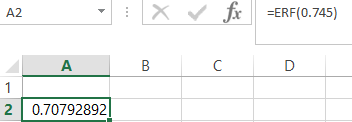Explanation: The Erf function integrated between 0 and 0.745000 is 0.707929

Engineering functions can be used to perform a wide range of engineering-related calculations, finding the error functions, and performing hyperbolic trigonometry calculations. To use these functions, simply enter the function name followed by the appropriate arguments in a cell in Excel, and then press the Enter key to get the result.